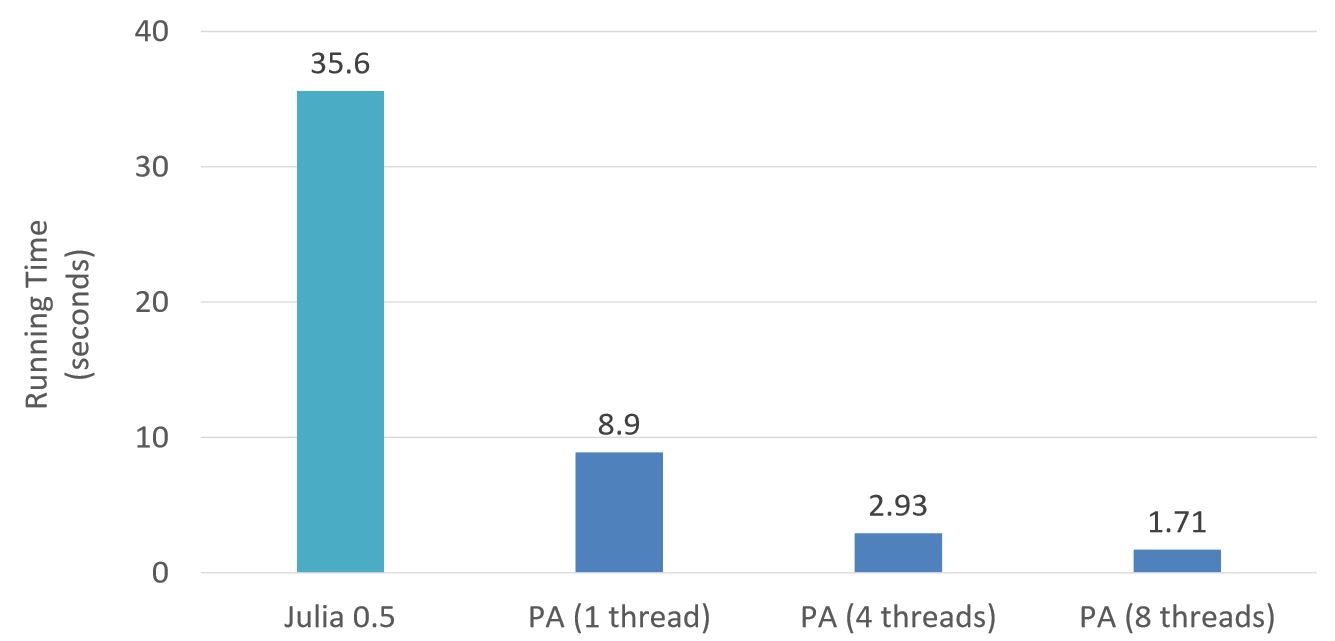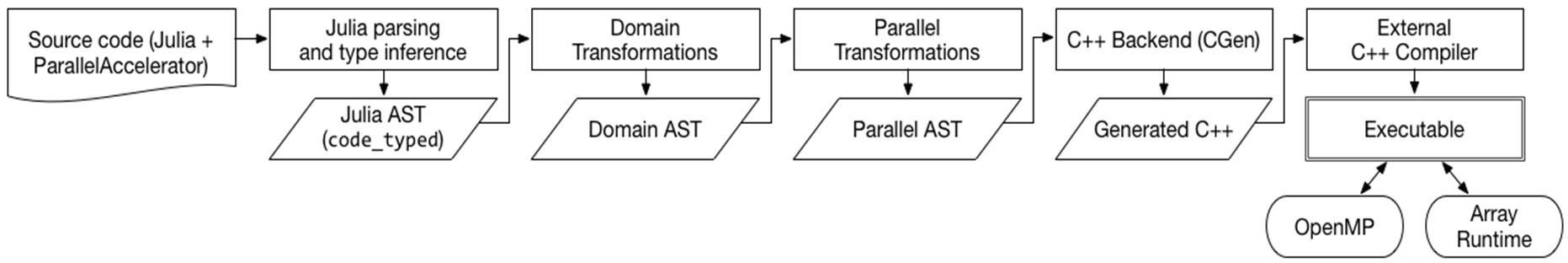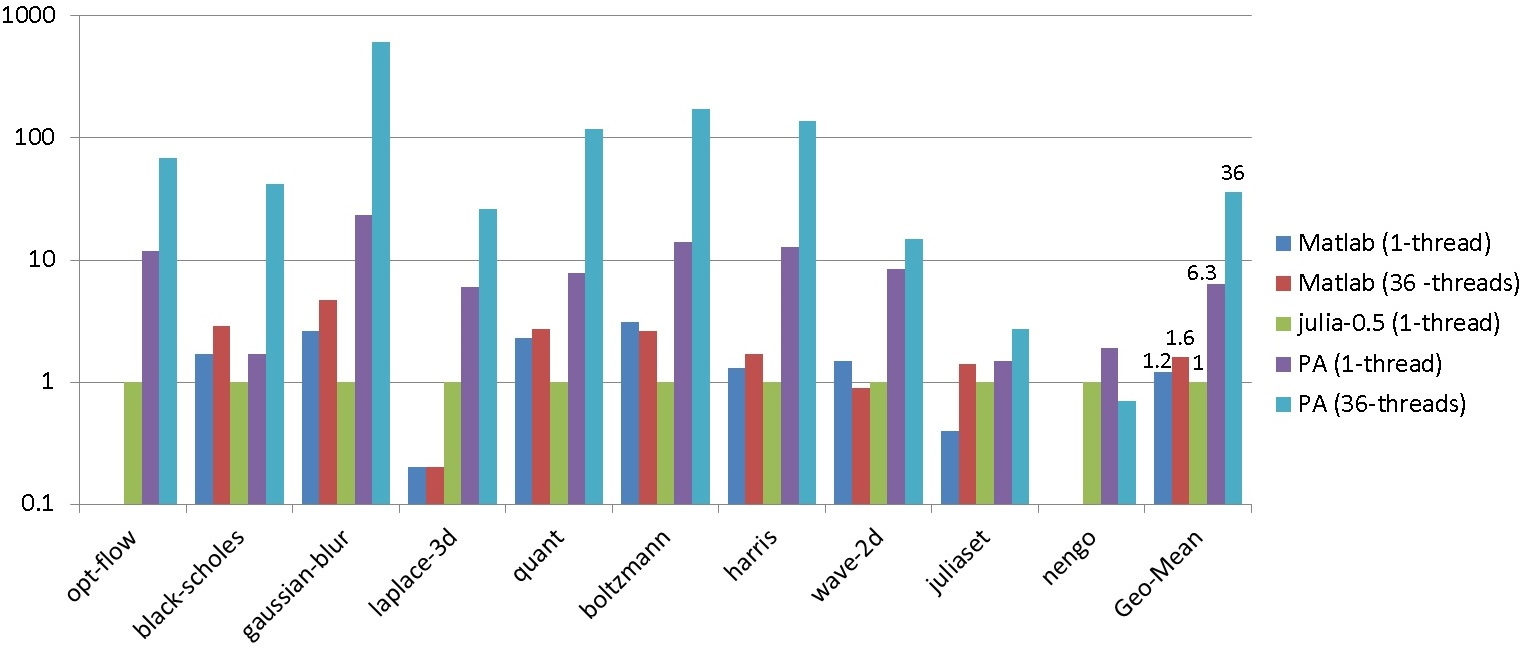# Productivity languages are great for prototyping

## Matlab, Python, R, …

Safe.

Dynamically typed.

Interactive dev environment.

Thriving eco-system.

## Option 1: re-implement in an HPC language (Fortran/C/C++)

Fast? Maybe.

Low-level, hard to use.

Hard to debug.

Hard to maintain.

Demands skills not possessed by domain experts.

## Option 2: Use a high performance library

Bindings to low-level libraries such as OpenBLAS, MKL, …

Good performance.

Easy to use.

Restricted by interface.

Not cache friendly.

Library calls do not compose in parallel.

## Option 3: Use a DSL (and its compiler)

High-level abstraction with domain semantics.

Compiled and optimized for performance.

Learning curve.

Hard to debug.

Limited eco-system.

# Julia: a dynamic language for numerical computing

Matlab-like syntax.

Powerful macros.

Expressive type system.

Performance via LLVM JIT.

# K-Means example

``````

# D: number of dimensions
# N: number of points
# C: number of centers
function kmeans(C, iterations, pts)
D,N = size(pts)
centers = rand(D, C)
for l in 1:iterations
dists = [sqrt(sum((pts[:,i]-centers[:,j])^2)) for j in 1:C, i in 1:N]
labels = [indmin(dists[:,i]) for i in 1:N]
centers = [sum(pts[j,labels.==i])/sum(labels.==i) for j in 1:D, i in 1:C]
end
return centers
end``````
``````

# D: number of dimensions
# N: number of points
# C: number of centers
function kmeans(C, iterations, pts)
D,N = size(pts)
centers = rand(D, C)
for l in 1:iterations
dists = [sqrt(sum((pts[:,i]-centers[:,j])^2)) for j in 1:C, i in 1:N]
labels = [indmin(dists[:,i]) for i in 1:N]
centers = [sum(pts[j,labels.==i])/sum(labels.==i) for j in 1:D, i in 1:C]
end
return centers
end``````
``````

# D: number of dimensions
# N: number of points
# C: number of centers
function kmeans(C, iterations, pts)
D,N = size(pts)
centers = rand(D, C)
for l in 1:iterations
dists = [sqrt(sum((pts[:,i]-centers[:,j])^2)) for j in 1:C, i in 1:N]
labels = [indmin(dists[:,i]) for i in 1:N]
centers = [sum(pts[j,labels.==i])/sum(labels.==i) for j in 1:D, i in 1:C]
end
return centers
end``````
``````

# D: number of dimensions
# N: number of points
# C: number of centers
function kmeans(C, iterations, pts)
D,N = size(pts)
centers = rand(D, C)
for l in 1:iterations
dists = [sqrt(sum((pts[:,i]-centers[:,j])^2)) for j in 1:C, i in 1:N]
labels = [indmin(dists[:,i]) for i in 1:N]
centers = [sum(pts[j,labels.==i])/sum(labels.==i) for j in 1:D, i in 1:C]
end
return centers
end``````
``````

# D: number of dimensions
# N: number of points
# C: number of centers
function kmeans(C, iterations, pts)
D,N = size(pts)
centers = rand(D, C)
for l in 1:iterations
dists = [sqrt(sum((pts[:,i]-centers[:,j])^2)) for j in 1:C, i in 1:N]
labels = [indmin(dists[:,i]) for i in 1:N]
centers = [sum(pts[j,labels.==i])/sum(labels.==i) for j in 1:D, i in 1:C]
end
return centers
end``````
``````

# D: number of dimensions
# N: number of points
# C: number of centers
function kmeans(C, iterations, pts)
D,N = size(pts)
centers = rand(D, C)
for l in 1:iterations
dists = [sqrt(sum((pts[:,i]-centers[:,j])^2)) for j in 1:C, i in 1:N]
labels = [indmin(dists[:,i]) for i in 1:N]
centers = [sum(pts[j,labels.==i])/sum(labels.==i) for j in 1:D, i in 1:C]
end
return centers
end``````
``````using ParallelAccelerator

# D: number of dimensions
# N: number of points
# C: number of centers
@acc function kmeans(C, iterations, pts)
D,N = size(pts)
centers = rand(D, C)
for l in 1:iterations
dists = [sqrt(sum((pts[:,i]-centers[:,j])^2)) for j in 1:C, i in 1:N]
labels = [indmin(dists[:,i]) for i in 1:N]
centers = [sum(pts[j,labels.==i])/sum(labels.==i) for j in 1:D, i in 1:C]
end
return centers
end``````

## On steroids!# ParallelAccelerator

A non-invasive DSL compiler for multi-core.

Written in Julia.

Optimizes a subset of Julia language and library calls.

Compiles to C++ with OpenMP (or LLVM with Julia threads).

## What is optimized and parallelized

Array operators/functions.

Comprehension.

Stencil (`runStencil`).

Parallel for-loop (`@par`).

## Compilation Pipeline``````
dists = [sqrt(sum((pts[:,i]-centers[:,j])^2)) for j in 1:C, i in 1:N]
``````
``````
dists = [sqrt(sum((pts[:,i]-centers[:,j])^2)) for j in 1:C, i in 1:N]
# Domain IR

select(pts, :, i), select(centers, :, j)

``````
``````
dists = [sqrt(sum((pts[:,i]-centers[:,j])^2)) for j in 1:C, i in 1:N]
# Domain IR

map(-, select(pts, :, i), select(centers, :, j))

``````
``````
dists = [sqrt(sum((pts[:,i]-centers[:,j])^2)) for j in 1:C, i in 1:N]
# Domain IR

map(x->x^2,
map(-, select(pts, :, i), select(centers, :, j)))

``````
``````
dists = [sqrt(sum((pts[:,i]-centers[:,j])^2)) for j in 1:C, i in 1:N]
# Domain IR

reduce(+, 0,
map(x->x^2,
map(-, select(pts, :, i), select(centers, :, j))))

``````
``````
dists = [sqrt(sum((pts[:,i]-centers[:,j])^2)) for j in 1:C, i in 1:N]
# Domain IR

sqrt(reduce(+, 0,
map(x->x^2,
map(-, select(pts, :, i), select(centers, :, j)))))

``````
``````
dists = [sqrt(sum((pts[:,i]-centers[:,j])^2)) for j in 1:C, i in 1:N]
# Domain IR
catesianarray((i, j) ->
sqrt(reduce(+, 0,
map(x->x^2,
map(-, select(pts, :, i), select(centers, :, j))))),
(C, N))
``````
``````
dists = [sqrt(sum((pts[:,i]-centers[:,j])^2)) for j in 1:C, i in 1:N]
# Domain IR
dists = catesianarray((i, j) ->
sqrt(reduce(+, 0,
map(x->x^2,
map(-, select(pts, :, i), select(centers, :, j))))),
(C, N))
``````
``````
dists = [sqrt(sum((pts[:,i]-centers[:,j])^2)) for j in 1:C, i in 1:N]
# Domain IR
dists = catesianarray((i, j) ->
sqrt(reduce(+, 0,
map(x->x^2,
map(-, select(pts, :, i), select(centers, :, j))))),
(C, N))
# Parallel IR (after fusion)

parfor k in 1:D
x = pts[k,i] - centers[k,j]

end

``````
``````
dists = [sqrt(sum((pts[:,i]-centers[:,j])^2)) for j in 1:C, i in 1:N]
# Domain IR
dists = catesianarray((i, j) ->
sqrt(reduce(+, 0,
map(x->x^2,
map(-, select(pts, :, i), select(centers, :, j))))),
(C, N))
# Parallel IR (after fusion)

parfor k in 1:D
x = pts[k,i] - centers[k,j]
y = x^2
end

``````
``````
dists = [sqrt(sum((pts[:,i]-centers[:,j])^2)) for j in 1:C, i in 1:N]
# Domain IR
dists = catesianarray((i, j) ->
sqrt(reduce(+, 0,
map(x->x^2,
map(-, select(pts, :, i), select(centers, :, j))))),
(C, N))
# Parallel IR (after fusion)

s = 0
parfor k in 1:D
x = pts[k,i] - centers[k,j]
s += x^2
end

``````
``````
dists = [sqrt(sum((pts[:,i]-centers[:,j])^2)) for j in 1:C, i in 1:N]
# Domain IR
dists = catesianarray((i, j) ->
sqrt(reduce(+, 0,
map(x->x^2,
map(-, select(pts, :, i), select(centers, :, j))))),
(C, N))
# Parallel IR (after fusion)

s = 0
parfor k in 1:D
x = pts[k,i] - centers[k,j]
s += x^2
end
z = sqrt(s)

``````
``````
dists = [sqrt(sum((pts[:,i]-centers[:,j])^2)) for j in 1:C, i in 1:N]
# Domain IR
dists = catesianarray((i, j) ->
sqrt(reduce(+, 0,
map(x->x^2,
map(-, select(pts, :, i), select(centers, :, j))))),
(C, N))
# Parallel IR (after fusion)
dists = array(float64, C, N)
parfor i in 1:C, j in 1:N
s = 0
parfor k in 1:D
x = pts[k,i] - centers[k,j]
s += x^2
end
dists[i,j] = sqrt(s)
end
``````

## Switch-on performance

Same program.

Run in parallel with `@acc`.

Or run sequentially without.

### Optimizations

Size inference, loop fusion, allocation hoisting, SIMD vectorization, …

# Evaluation (speedups)# Take Away

## A non-invasive DSL for parallel computing

Easy to use like a library.

Heavily optimized like a DSL.

Good performance on multi-core.

Flexibility is both a blessing and a curse.

Performance tuning still requires research.

Julia’s macro system and type inference are key enablers.

# Thank you!

https://github.com/IntelLabs/ParallelAccelerator.jl